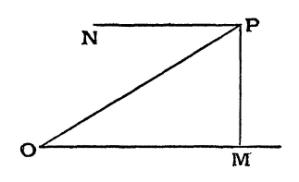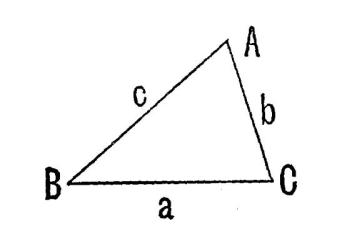The Learning Point‎ > ‎Mathematics‎ > ‎

## Trigonometry Tutorials: At a glanceTrigonometry 1a ( Introduction to Trigonometry - Definitions, Formulas )Trigonometry 1b ( Problems based on Trigonometric ratios )Trigonometry 2a ( Basic concepts related to Heights and Distances )Trigonometry 2b ( Problems on Heights, Distances and applications Trigonometry 3a ( Introducing Inverse Trigonometric Ratios)Trigonometry 3b (Problems related to inverse trigonometric ratios Trigonometry 4 ( A tutorial on solving trigonometric equations

------------------xxx---------------

## Heights and Distances, Properties of Triangles - Circumcircles, Incircles, Escribed Circles, Sine and Cosine Rules

### Target Audience: High School Students, College Freshmen and Sophomores, Class 11/12 Students in India preparing for ISC/CBSE and Entrance Examinations like the IIT-JEE, Anyone else who needs this Tutorial as a reference!

Here's a quick peek into what this tutorial introduces :

Important points to remember:
• The angle MOP is called the Angle of Elevation of the point P as seen from O. The angle NPO is the Angle of Depression of the point O as seen from P.• In any triangle ABC,• Sine formula: sin A/a =sin B/b=sin C/c=2/R, where R = Circum-radius

i.e. the sines of the angles are proportional to the opposite sides.

• Cosine formula : cos C = (a2+b2-c2)2ab, cos B = (a2+c2-b22ac, cosA = (c2+b2-a22cb

• sine, cosine and tangent of half angles:
• sin(A/2)= ( (s-b)(s-c) / (bc)) 0.5, where s=a+b+c2=semi-perimeter of a triangle
• cos(A/2)= ( (s)(s-a) / (bc) )0.5
• tan(A/2)= ( (s-b)(s-c) / ((s)(s-a)) )0.5

• The sine of any angle of a triangle in terms of the sides (this will be shown in the tutorial) .
• a = b cos C + c cos B
• tan(B-C)/2=(b-c)/(b+c cotA/2)
• Area() of a given triangle: ∆=12casin B =12ab sin C = 12bc sin A=ss-as-b(s-c)

### The circle which passes through the angular points of a triangle ABC is called its circumcircleThe Circum-Radius RThe circle which can be inscribed within the triangle so as to touch each of the sides is called its incircle. Its radius will be denoted by r.Incircle and Calculating the In-radius

• The circle which touches all sides of the triangle internally.

### Escribed Circle

The circle which touches the side BC and the two sides AB and AC produced is called the escribed circle opposite the angle A. Its radius will be denoted by r 1. Similarly r 2 denotes the radius of the circle which touches the side CA and the two sides BC and BA produced. Also r 3 denotes the radius of the circle touching AB and the two sides CA and CB produced.
• r 1=S/(s-a)= s/ tan (A/2)=4R / sin(A/2)cos(B/2)cos(C/2)
• r 2=S/(s-b)
• r 3=S/(s-c)

### Orthocenter, Pedal Triangles, Medians and Centroid

• Let ABC be any triangle and let AK, BL, and CM be the perpendiculars from A, B, and C upon the opposite sides of the triangle. These three perpendiculars meet in a common point P. This point P is called the orthocentre of the triangle. The triangle KLM, which is formed by joining the feet of these perpendiculars, is called the pedal triangle of ABC.
• Distances of the orthocentre from different points of the triangle.
• PK=2RcosBcosC, PL = 2RcosAcosC, PM = 2RcosAcosB
• AP = 2RcosA, BP = 2R cos B, and CP = 2R cos C
• Sides and angles of the pedal triangle.
• LM = acosA, MK = b cos B, and KL = c cos C.
• Centroid and Medians of any Triangle if ABC be any triangle, and D, E, and F respectively the middle points of BC, CA, and AB, the lines AD, BE, and CF are called the medians of the triangle and the medians are concurrent at a point known as the Centroid of the triangle.

Complete Tutorial with Figures, Formulas, Examples, Problems and Solutions :